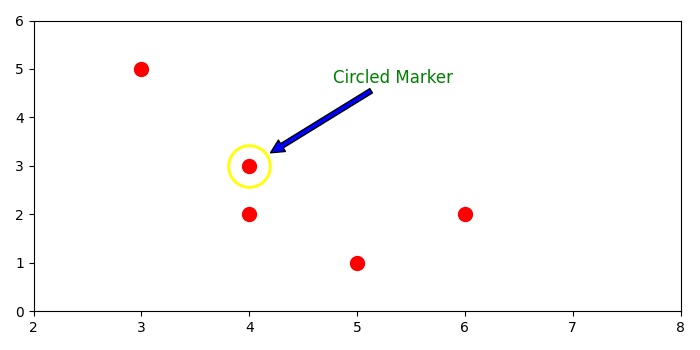# How do I put a circle with annotation in matplotlib?

To put a circle with annotation in matplotlib, we can take the following steps −

• Set the figure size and adjust the padding between and around the subplots.
• Create data points using numpy.
• Get the point coordinate to put circle with annotation.
• Get the current axis.
• Plot the data and data points using plot() method.
• Set X and Y axes scale.
• To put a circled marker, use the plot() method with marker='o' and some properties.
• Annotate that circle (Step 7) with arrow style.
• To display the figure, use show() method.

## Example

import matplotlib.pyplot as plt
import numpy as np

plt.rcParams["figure.figsize"] = [7.00, 3.50]
plt.rcParams["figure.autolayout"] = True

data = np.array([[5, 3, 4, 4, 6],
[1, 5, 3, 2, 2]])
point = data[:, 2]
ax = plt.gca()
ax.plot(data, data, 'o', ms=10, color='red')

ax.set_xlim([2, 8])
ax.set_ylim([0, 6])

ax.plot(point, point, 'o',
ms=radius * 2, mec='yellow', mfc='none', mew=2)

ax.annotate('Circled Marker', xy=point, xytext=(60, 60),
textcoords='offset points',
color='green', size='large',
arrowprops=dict(
plt.show()